# Image Formation in Convex Mirror

Image formation in a convex mirror depends mainly on two possibilities. It mainly deals with the position of an object in a convex mirror whether it is at infinity or when the object is between infinity and the pole of the mirror. However, before we talk more about the formation of image in a convex mirror we shall understand a few important topics.

## What is Convex Mirror?

A convex mirror is a type of spherical mirror in which the reflecting surface is the bulged out portion of the sphere, i.e. in this type of mirrors the reflecting surface bulges out towards the incident light source. Because of their shape the incident light is reflected outwards (diverged), thus they are also called diverging mirrors and thus they are not used for focusing light.

Both the laws of reflection are valid at each and every point on the mirror. The normal in this case is drawn normal to the tangent at the point of incidence, i.e. it is drawn by joining the centre of curvature of the mirror to the point of incidence. Thus, the divergence of the rays after reflection is because the normal to the surface differs at each point on the mirror.

Convex mirrors always form virtual images. This is because the focal point and the centre of curvature of the convex mirror are imaginary points and that cannot be reached. So the image is formed inside the mirror and cannot be projected on a screen. The image formed look smaller than the object from a distance but it gets larger as the object is brought closer to the mirror.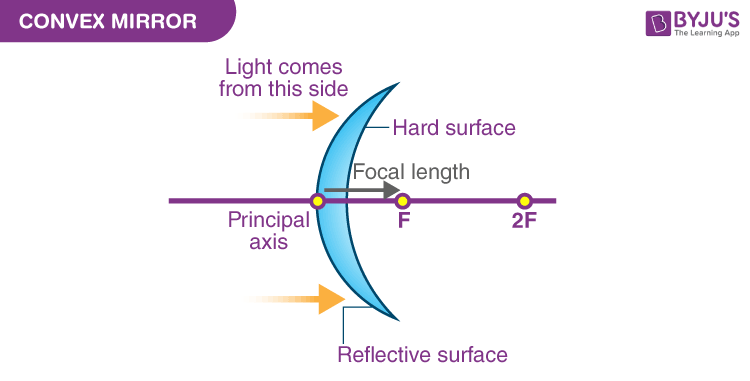## Some Important Terms

1. Pole: It is the centre of the reflecting surface of a spherical mirror. It lies on the surface of the mirror and it is usually denoted by P.

2. Centre of curvature: The centre of the sphere formed by the reflecting part of a spherical mirror is called the centre of curvature. It is generally denoted by C. this is not a part of the mirror and it lies outside the reflecting surface of the mirror. For a convex mirror, it lies behind the mirror.

Also Read: Mirror Formula For Spherical Mirrors

3. Radius of curvature: It is the radius of the sphere formed by the reflecting part of the sphere. It is represented by R.

4. Principal axis: It is the straight-line parring through the pole and centre of curvature of the spherical mirror. This is normal to the mirror at its pole.

5. Principal focus: The incident rays coming parallel to the principal axis after reflection appear to diverge from a common point on the principal axis, this point is called the principal focus of a convex mirror. It is usually denoted by F.

6. Focal length: It is the distance between the pole and the principal focus of the mirror. It is denoted by f.

## Ray Diagrams

Ray diagrams are necessary for understanding the formation of an image by a convex mirror. For constructing ray diagrams and to learn the image formation, we should consider at least two incident rays coming from the object.

The intersection of these two reflected rays gives the position of an image of the object. In case of a convex mirror any of the following four ray diagrams can be used for locating the image formed:

a) When the ray is parallel to the principal axis. After reflection, the rays appear to diverge from the principal focus of a convex mirror.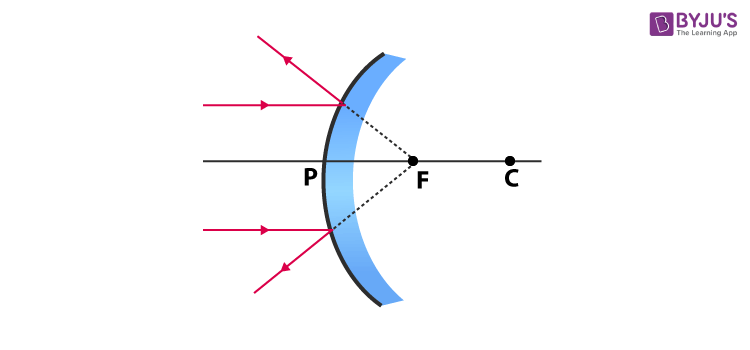b) When a ray is directed towards the principal focus of a convex mirror, after reflecting it will emerge parallel to the principal axis.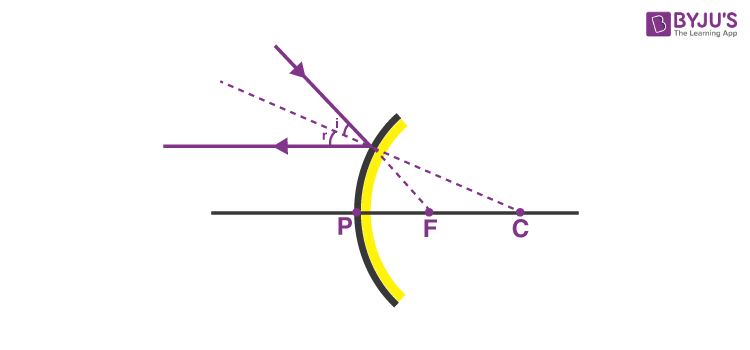c) When a ray is directed in the direction of the centre of curvature of a convex mirror the rays will be reflected along the same path. This occurs because the incident rays fall on the mirror along the normal to the reflecting surface.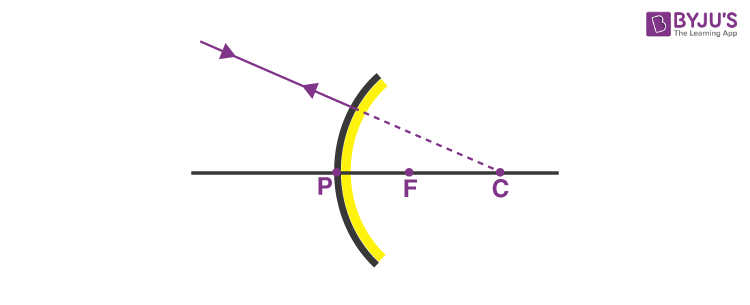d) When a ray incident is oblique to the principal axis and towards the pole of the mirror on the convex mirror, it is reflected obliquely. The incident and reflected rays follow the laws of reflection at point P, making equal angles with the principal axis.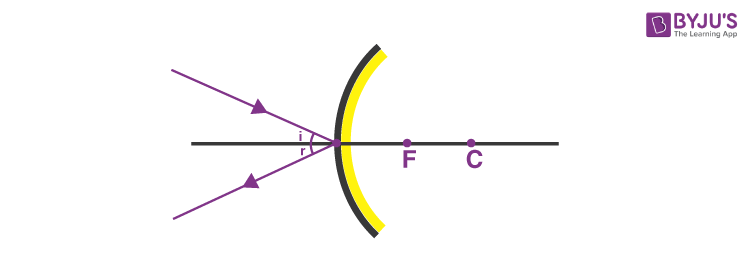## Image formation by Convex Mirror

Rays emerging from a point actually meet at another point after reflection, and that point is called the image of the first point. The image is real if the rays converge to the point and it is virtual if the rays do not meet it but appear to diverge from a point when the rays are produced backwards.

During image formation we assume that the rays are paraxial, i.e., they are incident at points close to the pole P of the mirror and make small angles with the principal axis. For a convex mirror, we consider two positions of the object before the mirror.

• When an object is placed at infinity.
• When the object is placed between infinity and the pole of the mirror.

For the image formation by a convex mirror in this condition, we consider two rays parallel to the principal axis and one directed towards the pole of the mirror originating from the object. These rays after reflection form an image at F, the principal focus of the mirror, which is behind the convex mirror. The image thus formed is highly diminished, virtual and erect.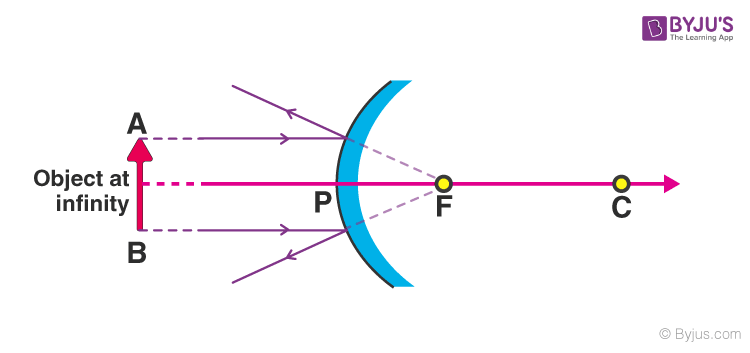### When The Object is Placed Between Infinity and Pole

In this situation of the image formation by a convex mirror, we consider two different rays emerging from the object. One parallel to the principal axis and the other directed towards the centre of curvature of the mirror. These rays after reflection form an image between the pole (P) and the focus (F) behind the mirror. The image thus formed is diminished, virtual and erect.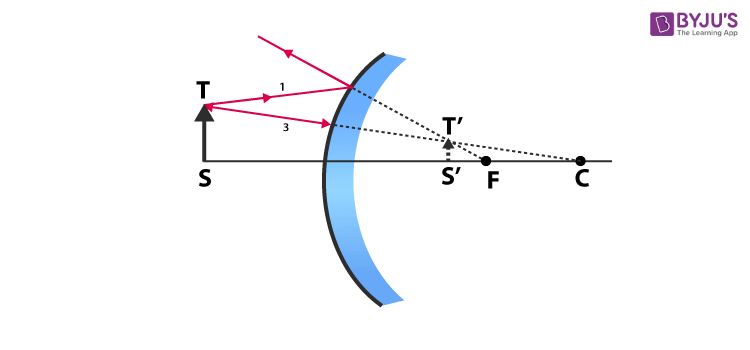Position of the object Position of the image Size of the image Nature of the image At infinity At focus, F, behind the mirror Highly diminished and pointed in size Virtual and erect Between infinity and pole of the mirror Between P and F, behind the mirror Diminished Virtual and erect

## Uses of Convex Mirrors

• Because they have wider fields of view, as they are curved outwards they are commonly used in automobile side mirrors.
• Convex mirrors are also a good security device because single security personnel could see large portions of the store while monitoring from a single location.
• They are used in driveways and roads, to provide safety to all the bikers and drivers at curves and other places where there is a lack of visibility.
• They are also used in ATMs as a handy security feature that allows the user to see what is happening behind them.
Test your Knowledge on Convex mirror